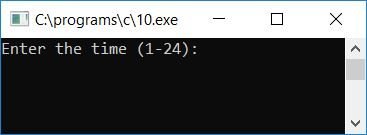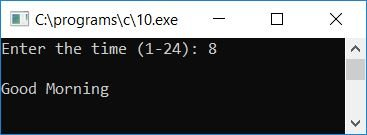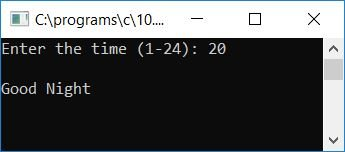# C Program to Print Good Morning, Evening, and Night According to Time

In this article, we will learn about how to create a program in C that takes time (in 24-hour format) as input from the user (at run-time) and prints a message accordingly, as defined below:

• If time is greater than 0 and less than or equal to 3, then print "Good Night."
• If the time is greater than 3 and less than 12, then print "Good Morning."
• If time is equal to 12, then print "Good Noon."
• If the time is greater than 12 and less than or equal to 15, print "Good Afternoon."
• If the time is more than 15 but less than 20, print "Good Evening."
• If time is greater than or equal to 20 and less than or equal to 24, "Good evening."

In 24-hour time format:

• If time equals 1, it is 1 a.m.
• If time equals 13, it is 1 p.m.
• If time equals 15, it is 3 p.m.
• If time equals 20, it is 8 p.m.
• and so no.

Let's take a look at the program:

```#include<stdio.h>
#include<conio.h>
int main()
{
int t;
printf("Enter the time (1-24): ");
scanf("%d", &t);
printf("\n");
if(t>0 && t<=3)
printf("Good Night");
else if(t>3 && t<12)
printf("Good Morning");
else if(t==12)
printf("Good Noon");
else if(t>12 && t<=15)
printf("Good AfterNoon");
else if(t>15 && t<20)
printf("Good Evening");
else if(t>=20 && t<=24)
printf("Good Night");
else
printf("Unknown time!");
getch();
return 0;
}```

Because the program was written in the Code::Blocks IDE, here is a snapshot of the sample run after a successful build and run:Now enter the time, say 8. The user has to enter the time in 24-hour format; entering 8 means 8 a.m., and entering 20 means 8 p.m. Here is the second snapshot of the sample run:Now let's check with another sample run by supplying 20 as input. In 24-hour format, 20 will be 8PM. Here is a snapshot of the second sample run:Below are some of the main steps used in the above program:

• Receive time (a number between 1 and 24) as input.
• Use an if-else statement to check and print the message accordingly.
• For example, if the user enters 13 as input, then 13 is greater than 12 and less than or equal to 15, therefore the 4th condition evaluates to true, and the message "Good Afternoon" gets printed on the output screen.
• If the user enters any time that is not between 1 and 24, then the program will output "Unknown time!"

C Quiz

« Previous Program Next Program »